Examples

Chapter 12 Class 10 Areas related to Circles
Serial order wise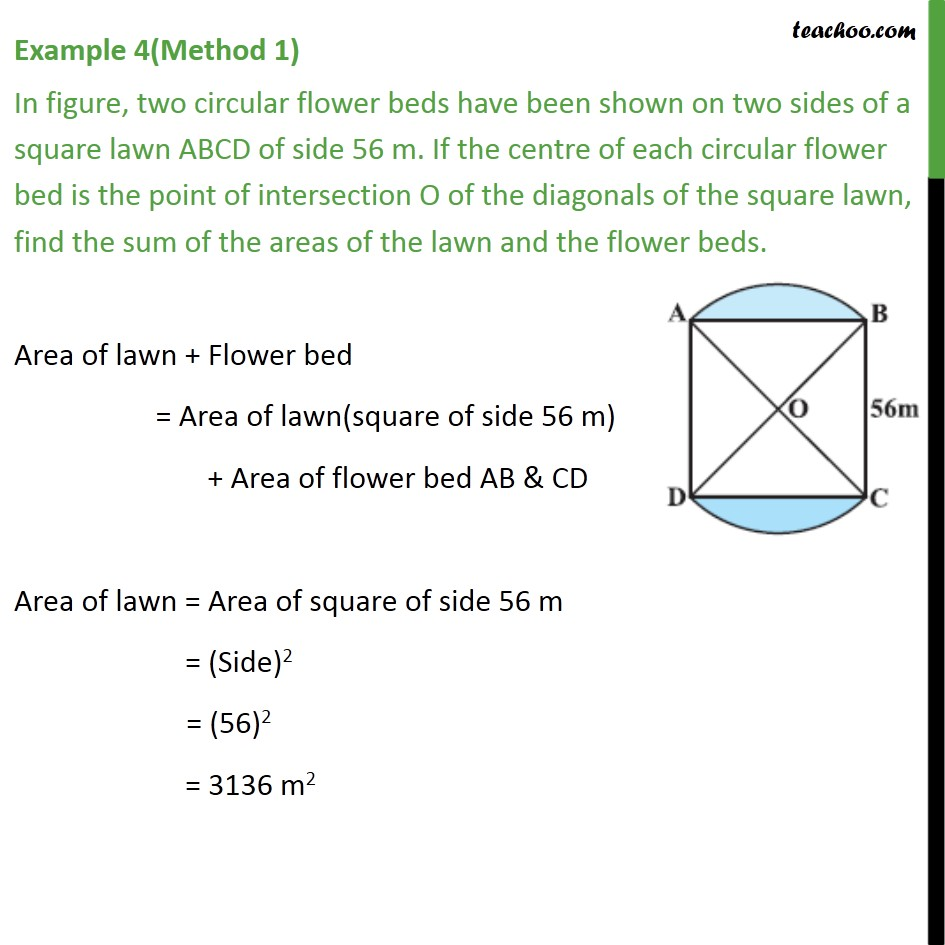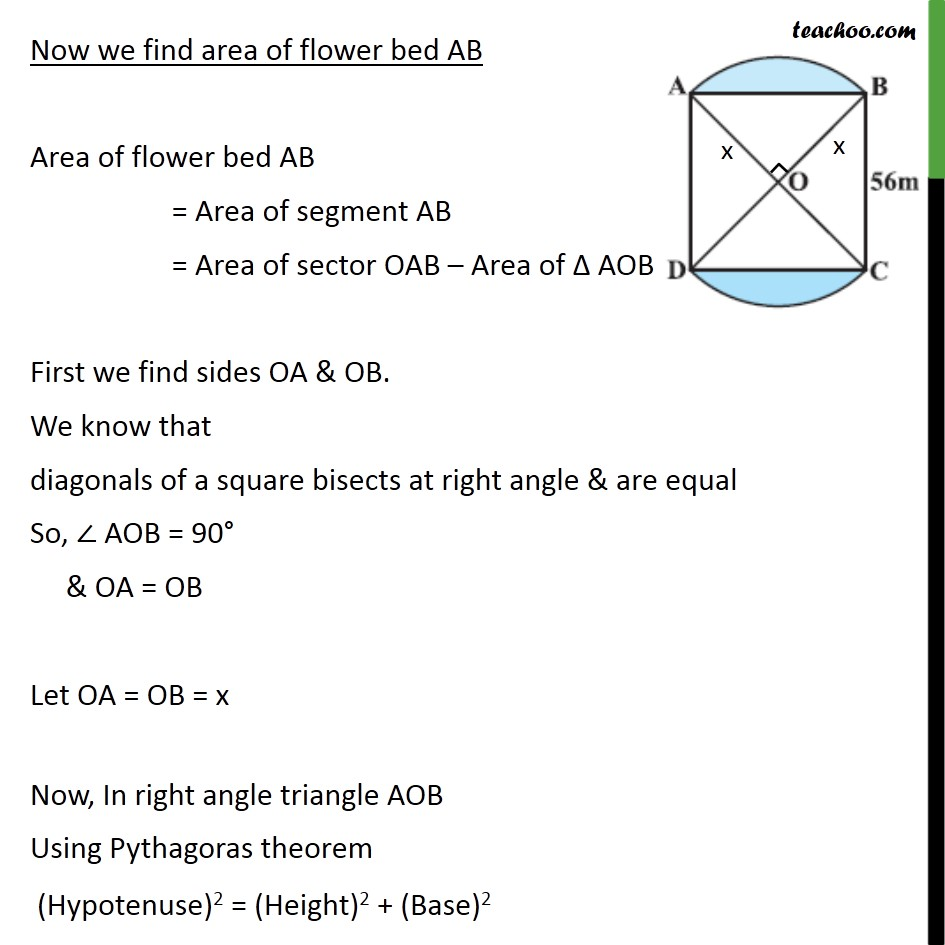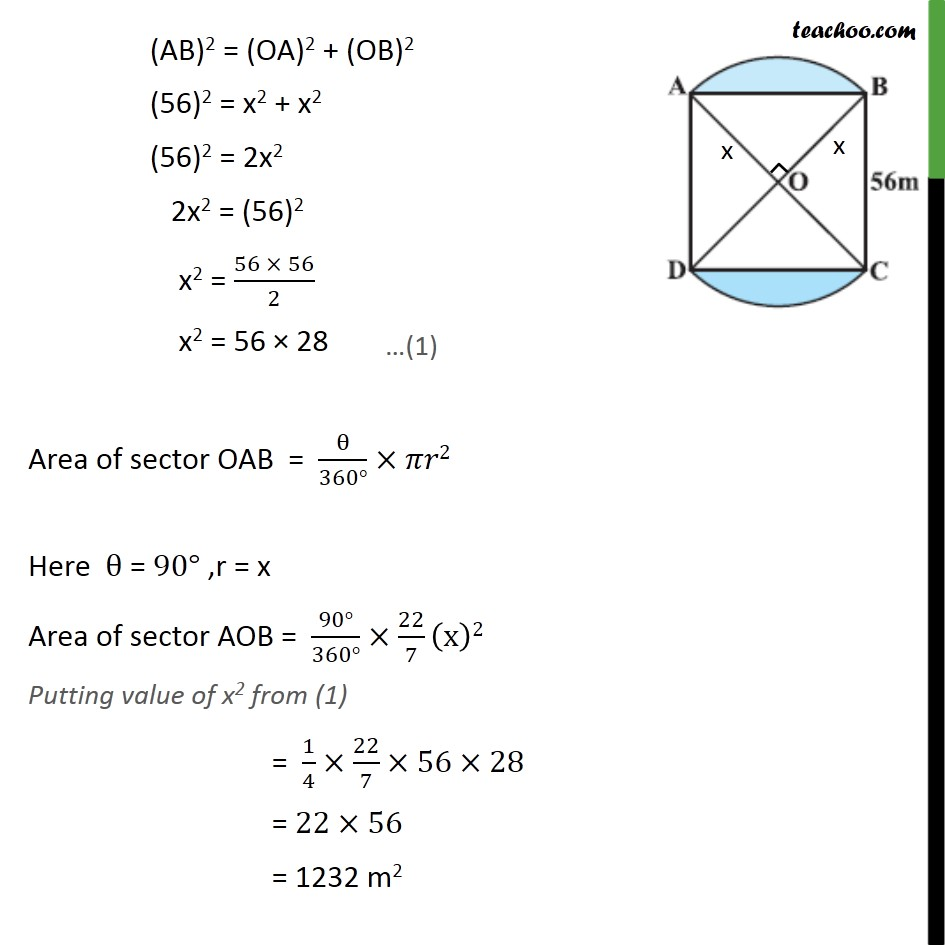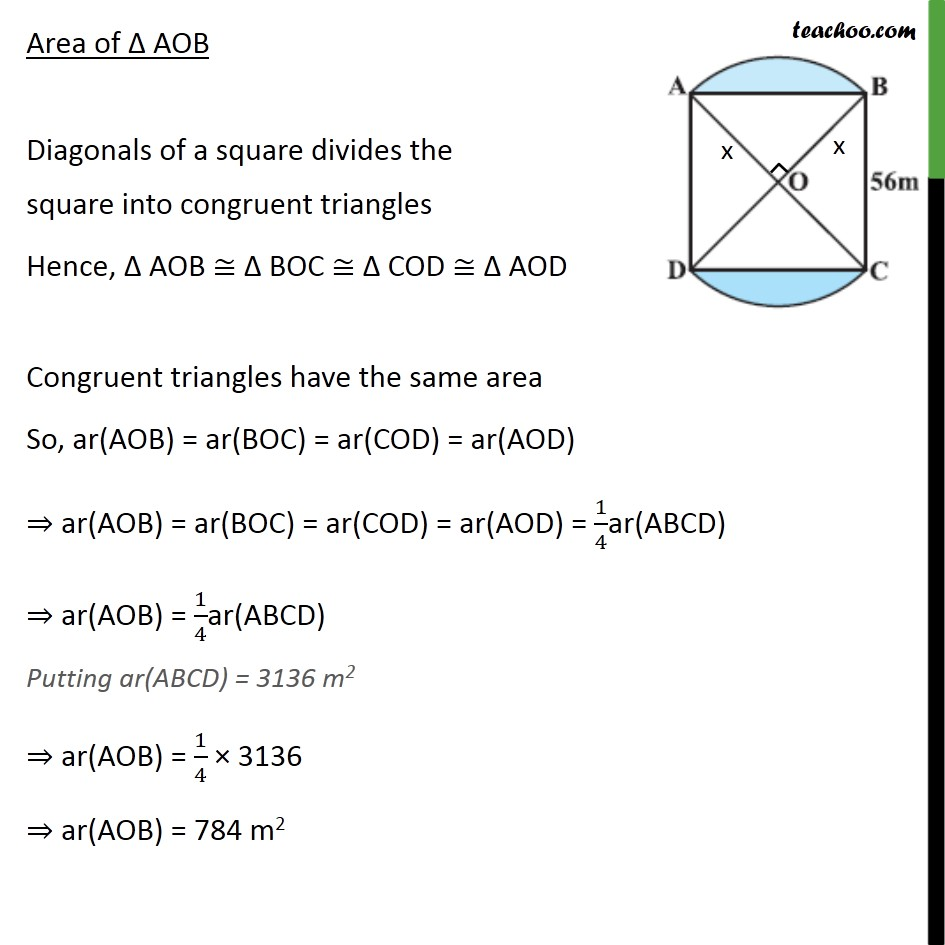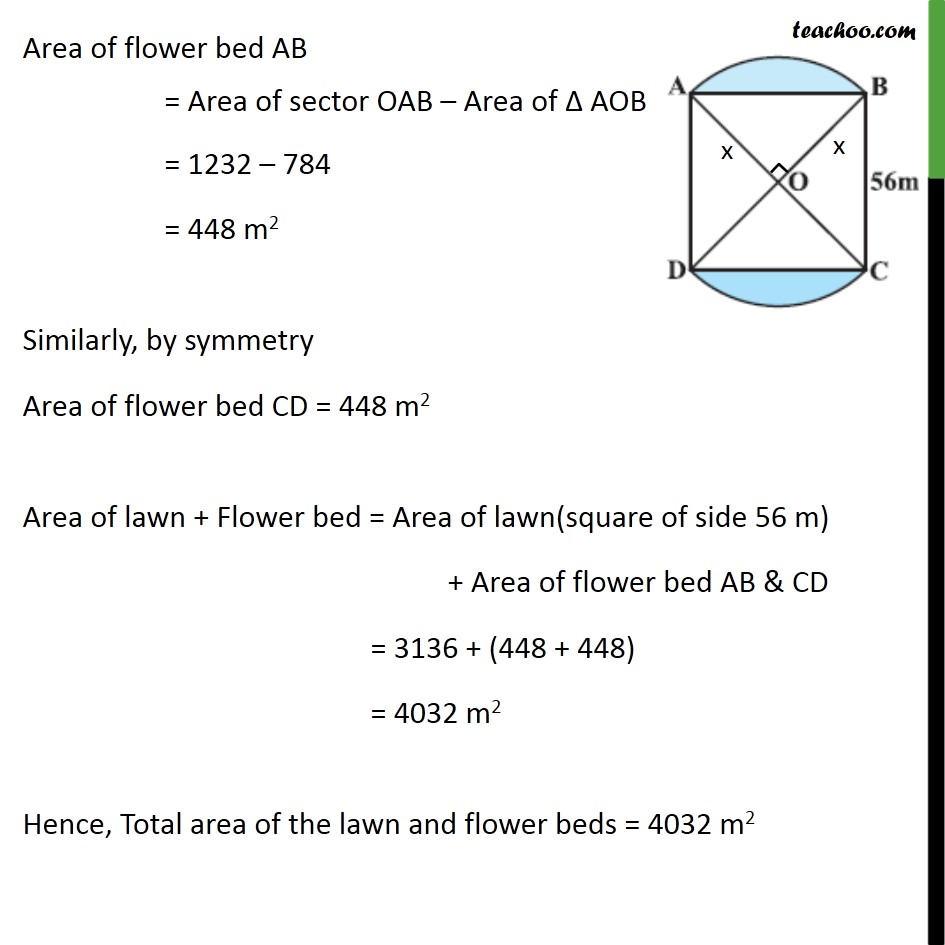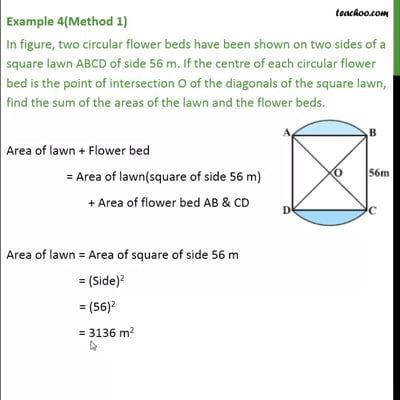This video is only available for Teachoo black users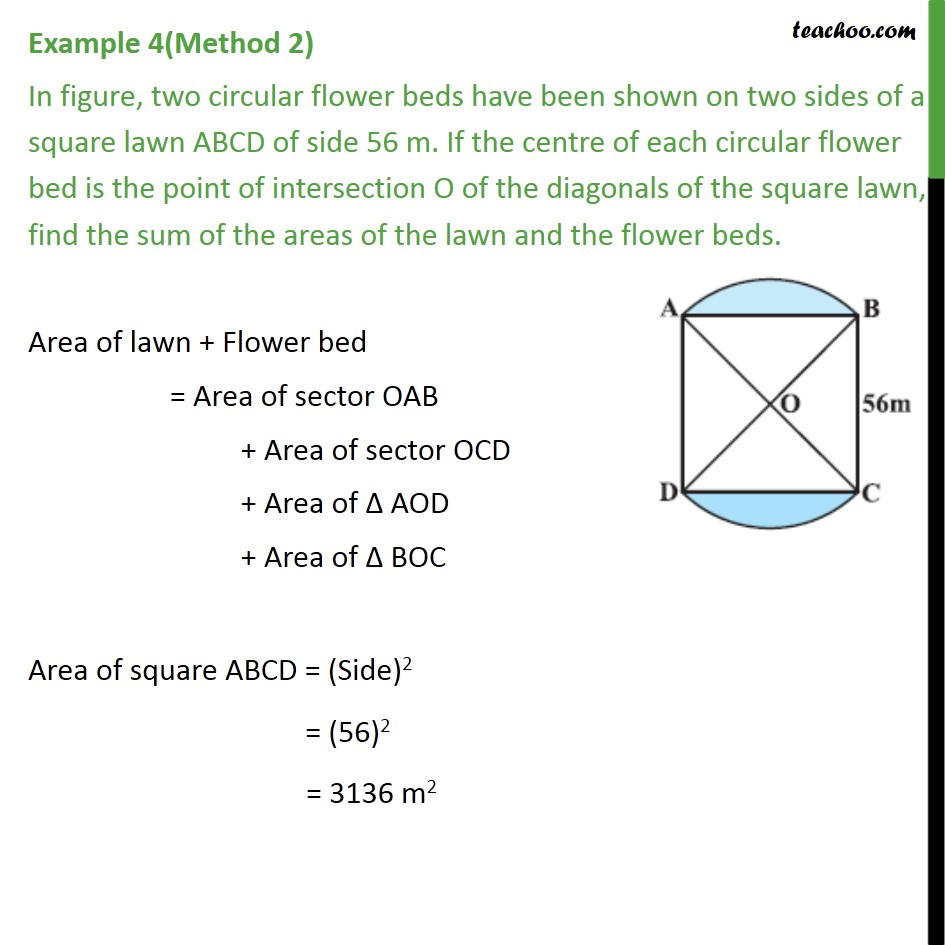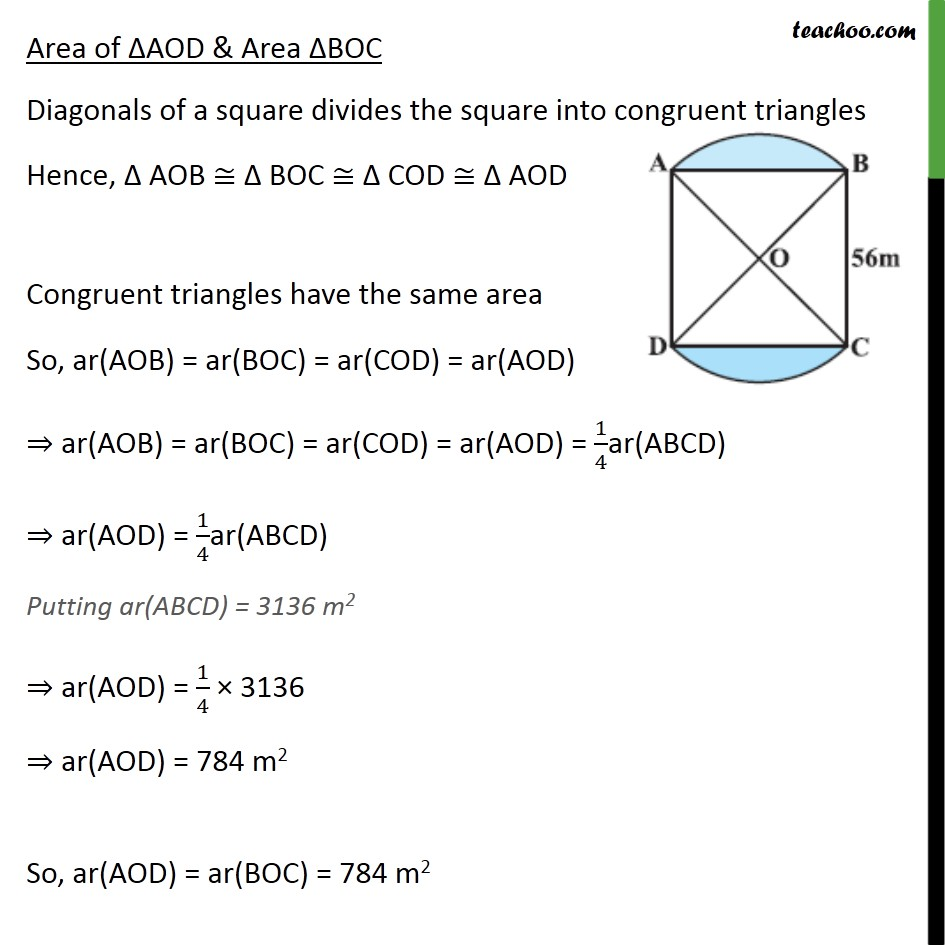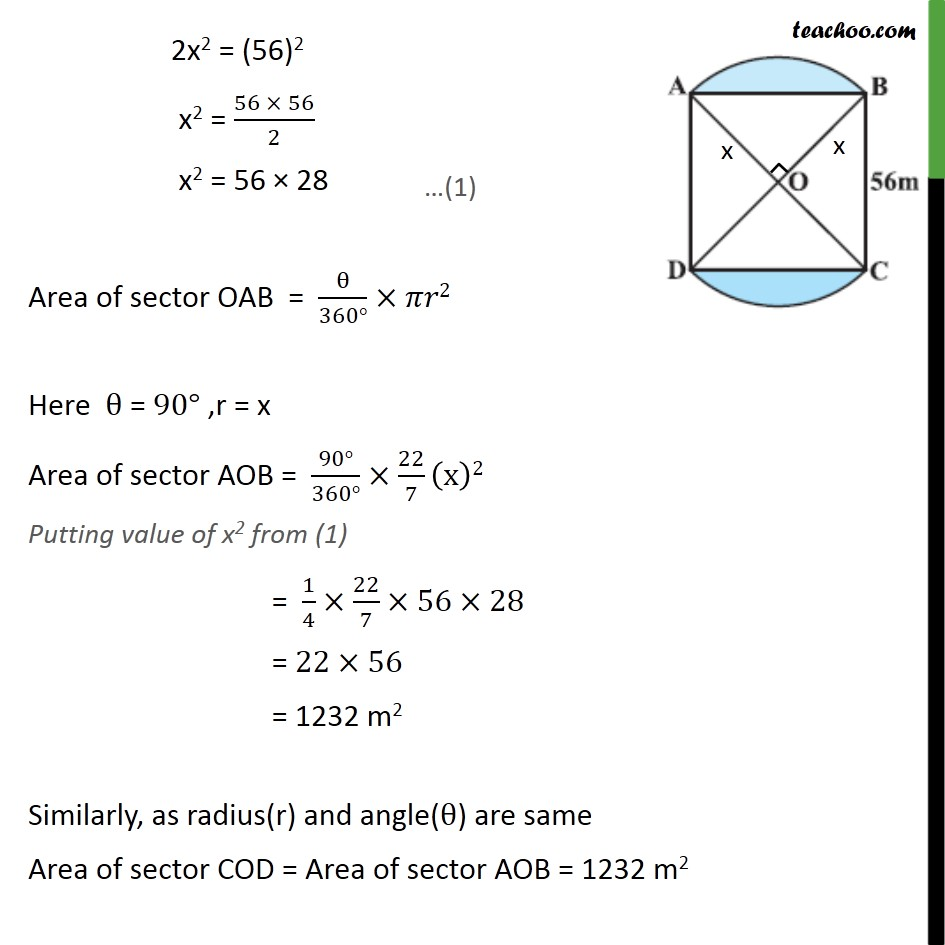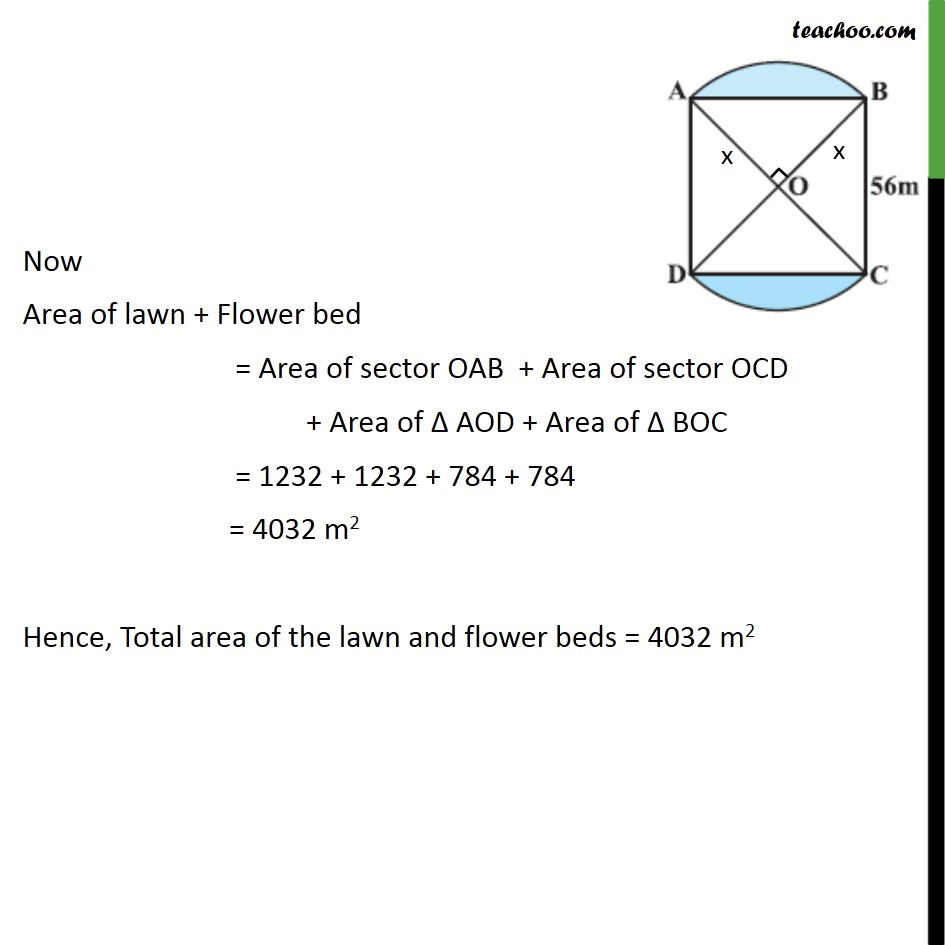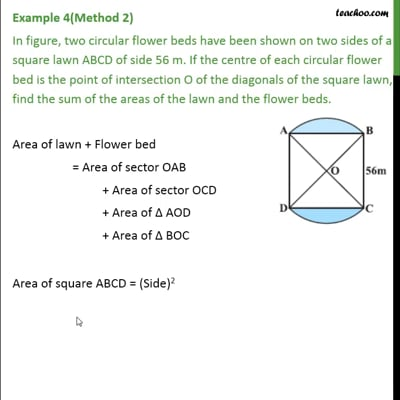This video is only available for Teachoo black users

Solve all your doubts with Teachoo Black (new monthly pack available now!)

### Transcript

Example 4(Method 1) In figure, two circular flower beds have been shown on two sides of a square lawn ABCD of side 56 m. If the centre of each circular flower bed is the point of intersection O of the diagonals of the square lawn, find the sum of the areas of the lawn and the flower beds. Area of lawn + Flower bed = Area of lawn(square of side 56 m) + Area of flower bed AB & CD Area of lawn = Area of square of side 56 m = (Side)2 = (56)2 = 3136 m2 Now we find area of flower bed AB Area of flower bed AB = Area of segment AB = Area of sector OAB – Area of Δ AOB First we find sides OA & OB. We know that diagonals of a square bisects at right angle & are equal So, ∠ AOB = 90° & OA = OB Let OA = OB = x Now, In right angle triangle AOB Using Pythagoras theorem (Hypotenuse)2 = (Height)2 + (Base)2 (AB)2 = (OA)2 + (OB)2 (56)2 = x2 + x2 (56)2 = 2x2 2x2 = (56)2 x2 = (56 × 56)/2 x2 = 56 × 28 Area of sector OAB = θ/(360°)×𝜋𝑟2 Here θ = 90° ,r = x Area of sector AOB = (90°)/(360°)×22/7 (x)2 Putting value of x2 from (1) = 1/4×22/7×56×28 = 22×56 = 1232 m2 Area of Δ AOB Diagonals of a square divides the square into congruent triangles Hence, Δ AOB ≅ Δ BOC ≅ Δ COD ≅ Δ AOD Congruent triangles have the same area So, ar(AOB) = ar(BOC) = ar(COD) = ar(AOD) ⇒ ar(AOB) = ar(BOC) = ar(COD) = ar(AOD) = 1/4ar(ABCD) ⇒ ar(AOB) = 1/4ar(ABCD) Putting ar(ABCD) = 3136 m2 ⇒ ar(AOB) = 1/4 × 3136 ⇒ ar(AOB) = 784 m2 Area of flower bed AB = Area of sector OAB – Area of Δ AOB = 1232 – 784 = 448 m2 Similarly, by symmetry Area of flower bed CD = 448 m2 Area of lawn + Flower bed = Area of lawn(square of side 56 m) + Area of flower bed AB & CD = 3136 + (448 + 448) = 4032 m2 Hence, Total area of the lawn and flower beds = 4032 m2 Example 4(Method 2) In figure, two circular flower beds have been shown on two sides of a square lawn ABCD of side 56 m. If the centre of each circular flower bed is the point of intersection O of the diagonals of the square lawn, find the sum of the areas of the lawn and the flower beds. Area of lawn + Flower bed = Area of sector OAB + Area of sector OCD + Area of Δ AOD + Area of Δ BOC Area of square ABCD = (Side)2 = (56)2 = 3136 m2 Area of ΔAOD & Area ΔBOC Diagonals of a square divides the square into congruent triangles Hence, Δ AOB ≅ Δ BOC ≅ Δ COD ≅ Δ AOD Congruent triangles have the same area So, ar(AOB) = ar(BOC) = ar(COD) = ar(AOD) ⇒ ar(AOB) = ar(BOC) = ar(COD) = ar(AOD) = 1/4ar(ABCD) ⇒ ar(AOD) = 1/4ar(ABCD) Putting ar(ABCD) = 3136 m2 ⇒ ar(AOD) = 1/4 × 3136 ⇒ ar(AOD) = 784 m2 So, ar(AOD) = ar(BOC) = 784 m2 Now we find area of sector OAB First we find sides OA & OB. We know that diagonals of a square bisects at right angle and are equal So, ∠ AOB = 90° & OA = OB Let OA = OB = x Now, In right angle triangle AOB Using Pythagoras theorem (Hypotenuse)2 = (Height)2 + (Base)2 (AB)2 = (OA)2 + (OB)2 (56)2 = x2 + x2 (56)2 = 2x2 2x2 = (56)2 x2 = (56 × 56)/2 x2 = 56 × 28 Area of sector OAB = θ/(360°)×𝜋𝑟2 Here θ = 90° ,r = x Area of sector AOB = (90°)/(360°)×22/7 (x)2 Putting value of x2 from (1) = 1/4×22/7×56×28 = 22×56 = 1232 m2 Similarly, as radius(r) and angle(θ) are same Area of sector COD = Area of sector AOB = 1232 m2 Now Area of lawn + Flower bed = Area of sector OAB + Area of sector OCD + Area of Δ AOD + Area of Δ BOC = 1232 + 1232 + 784 + 784 = 4032 m2 Hence, Total area of the lawn and flower beds = 4032 m2